Examples

Chapter 3 Class 12 Matrices
Serial order wise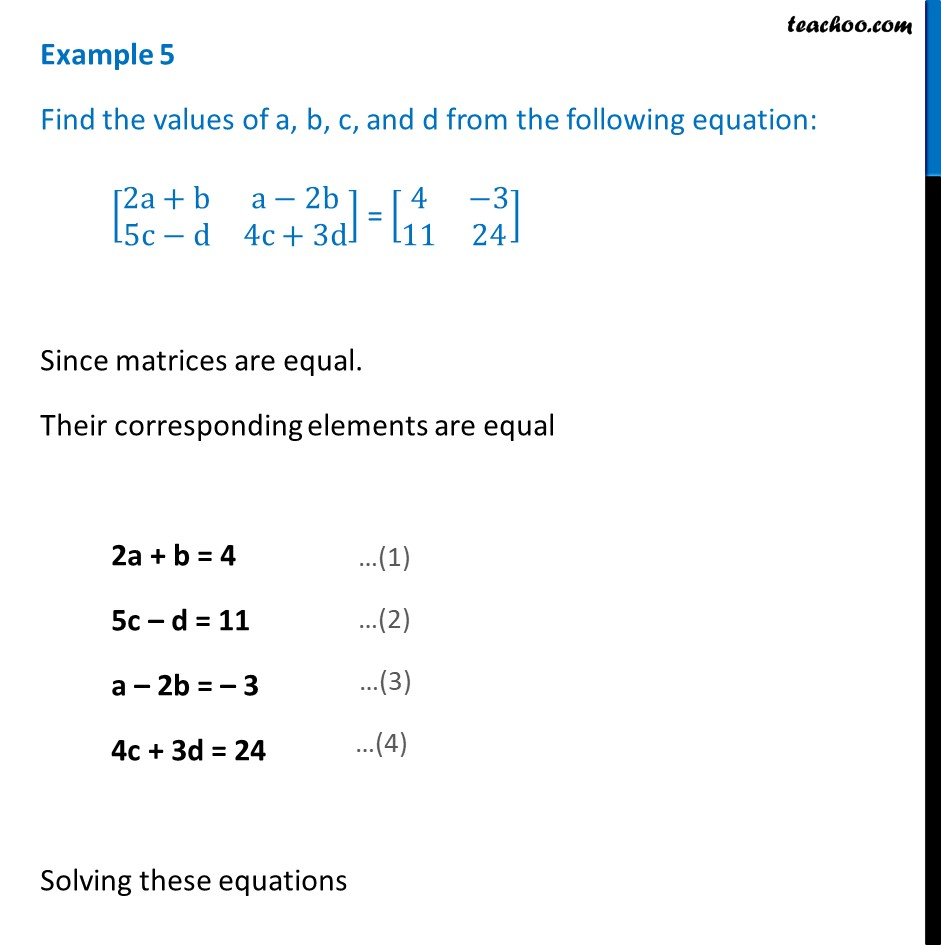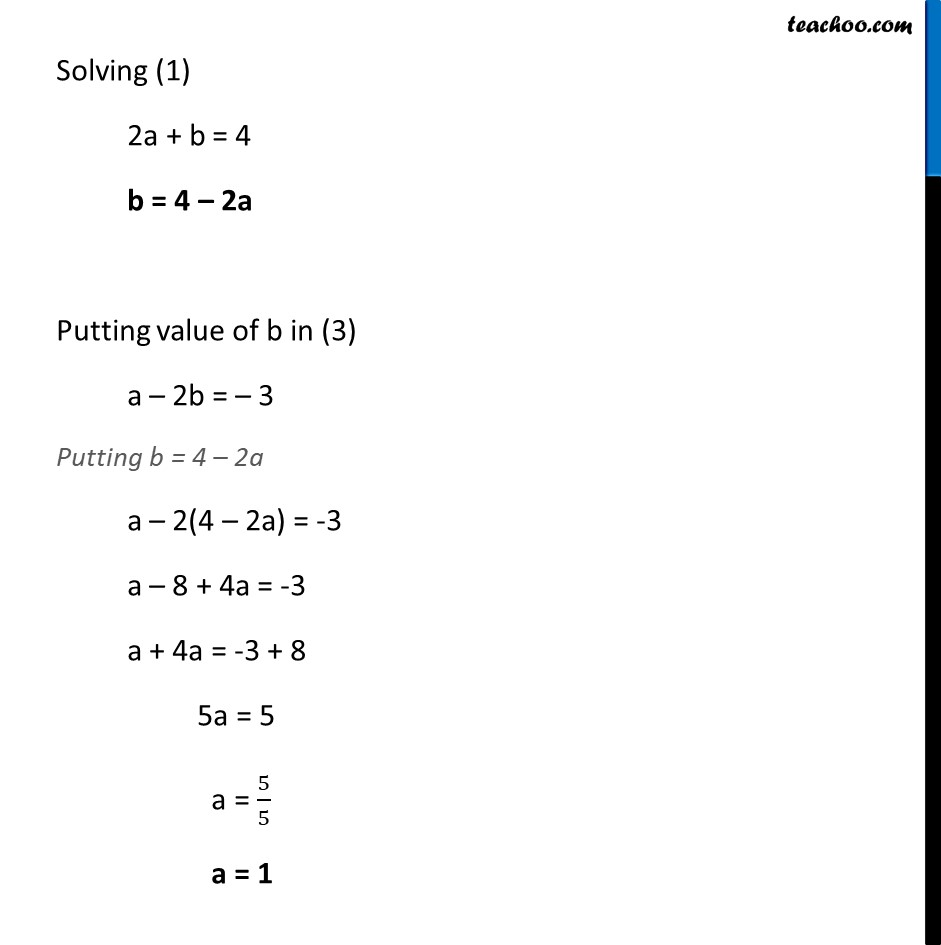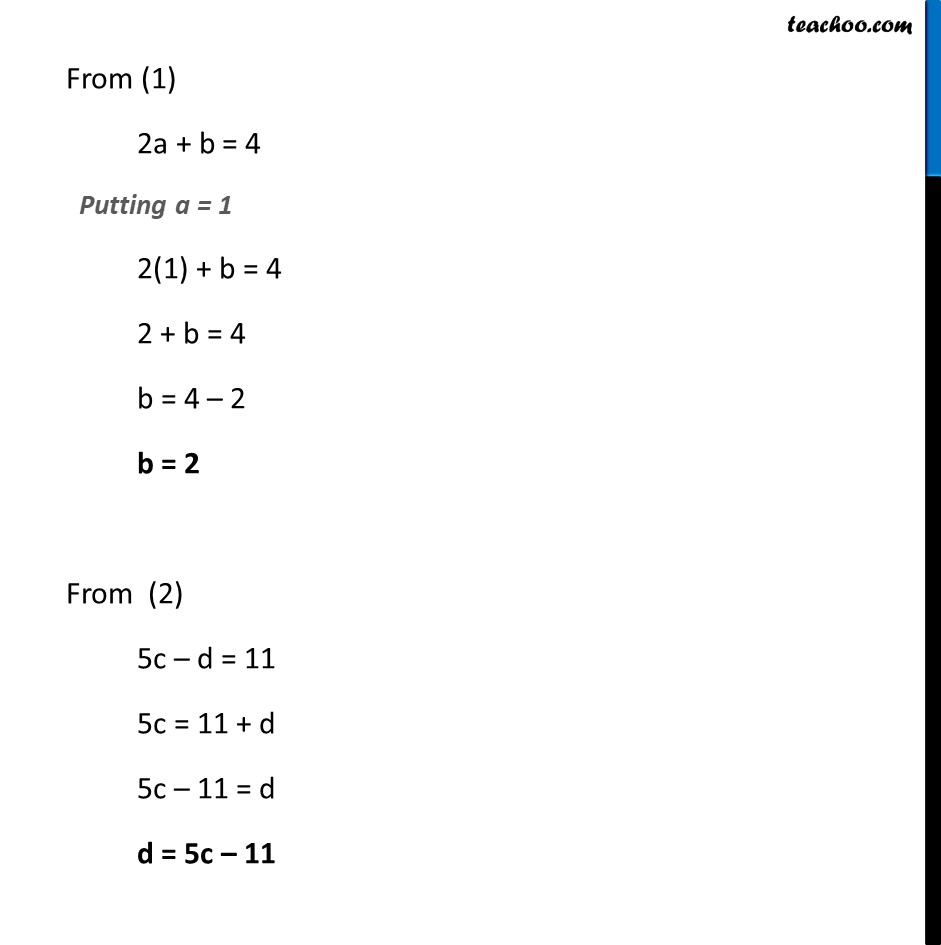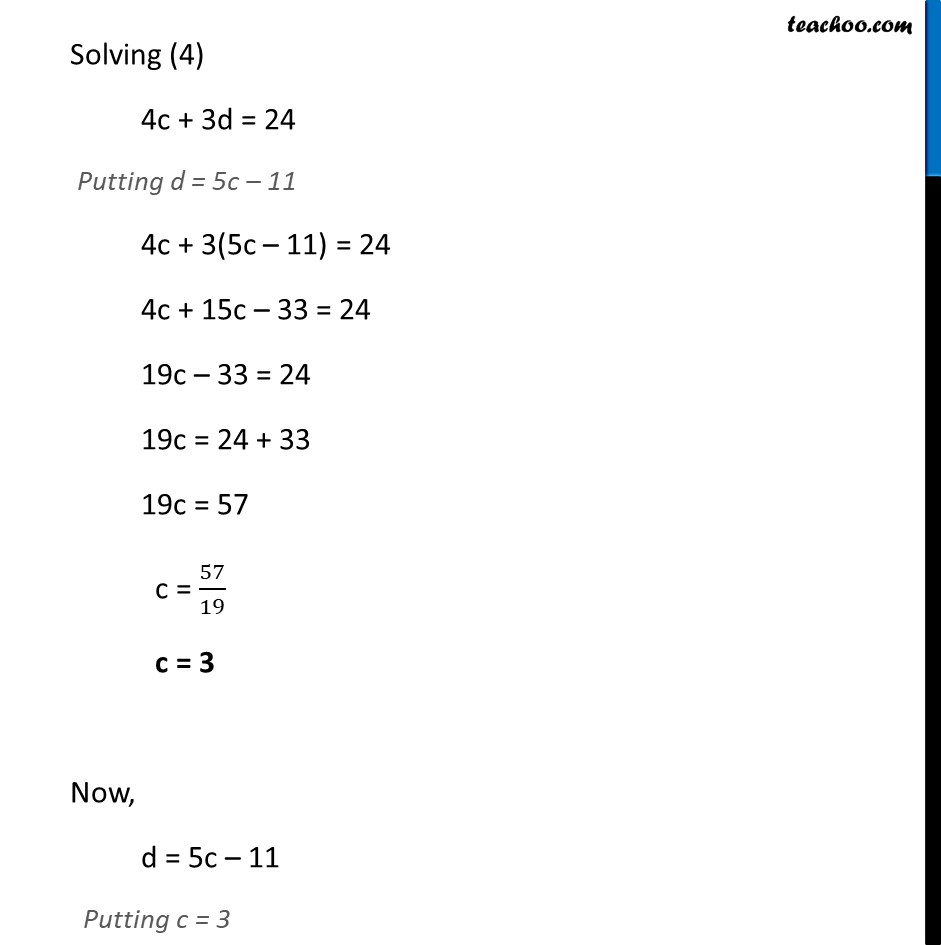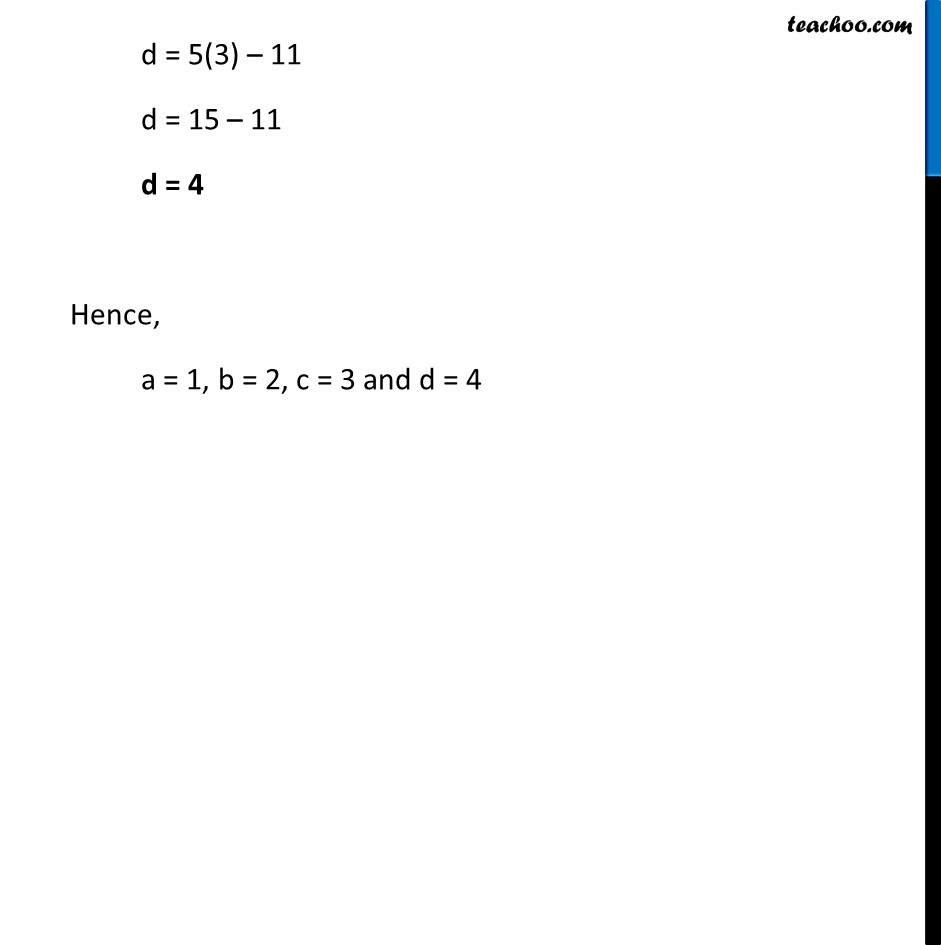Learn in your speed, with individual attention - Teachoo Maths 1-on-1 Class

### Transcript

Example 5 Find the values of a, b, c, and d from the following equation: [■8(2a+b&a−2b@5c−d&4c+3d)] = [■8(4&−3@11&24)] Since matrices are equal. Their corresponding elements are equal 2a + b = 4 5c – d = 11 a – 2b = – 3 4c + 3d = 24 Solving these equations Solving (1) 2a + b = 4 b = 4 – 2a Putting value of b in (3) a – 2b = – 3 Putting b = 4 – 2a a – 2(4 – 2a) = -3 a – 8 + 4a = -3 a + 4a = -3 + 8 5a = 5 a = 5/5 a = 1 From (1) 2a + b = 4 Putting a = 1 2(1) + b = 4 2 + b = 4 b = 4 – 2 b = 2 From (2) 5c – d = 11 5c = 11 + d 5c – 11 = d d = 5c – 11 Solving (4) 4c + 3d = 24 Putting d = 5c – 11 4c + 3(5c – 11) = 24 4c + 15c – 33 = 24 19c – 33 = 24 19c = 24 + 33 19c = 57 c = 57/19 c = 3 Now, d = 5c – 11 Putting c = 3 d = 5(3) – 11 d = 15 – 11 d = 4 Hence, a = 1, b = 2, c = 3 and d = 4